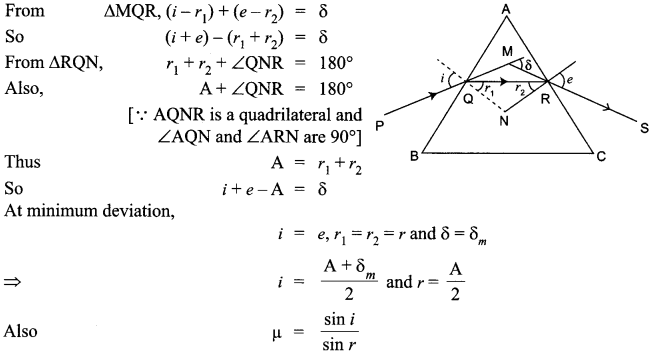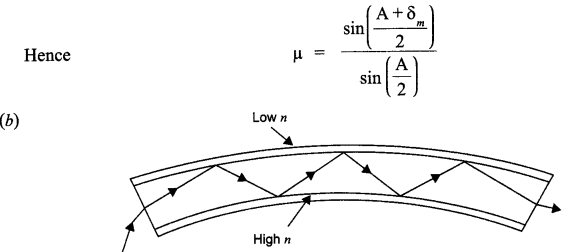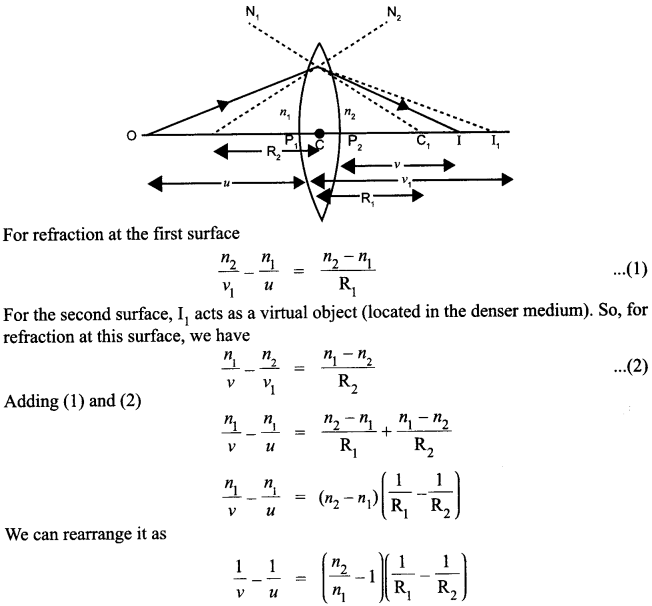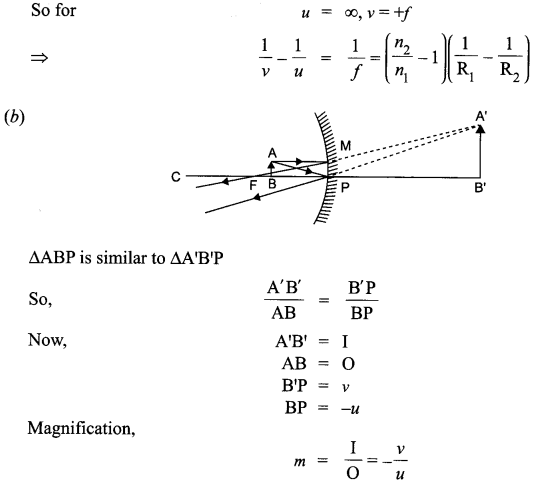CBSE Sample Papers for Class 12 Physics Paper 4 are part of CBSE Sample Papers for Class 12 Physics. Here we have given CBSE Sample Papers for Class 12 Physics Paper 4.

## CBSE Sample Papers for Class 12 Physics Paper 4

 Board CBSE Class XII Subject Physics Sample Paper Set Paper 4 Category CBSE Sample Papers
Students who are going to appear for CBSE Class 12 Examinations are advised to practice the CBSE sample papers given here which is designed as per the latest Syllabus and marking scheme as prescribed by the CBSE is given here. Paper 4 of Solved CBSE Sample Paper for Class 12 Physics is given below with free PDF download solutions.
Time Allowed : 3 Hours
Max. Marks : 70
General Instructions
• All questions are compulsory. There are 26 questions in all.
• This question paper has five sections: Section A, Section B, Section C, Section D and Section E.
• Section A contains five questions of 1 mark each. Section B contains five questions of 2 marks each. Section C contains twelve questions of 3 marks each. Section D contains one value based question of 4 marks and Section E contains three questions of 5 marks each.
• There is no overall choice. However, an internal choice has been provided in 1 question of 2 marks, 1 question of 3 marks and all the 3 questions of 5 marks weightage. You have to attempt only 1 of the choices in such questions.
• You may use the following values of physical constants wherever necessary :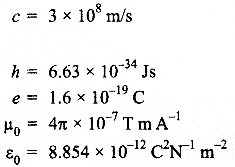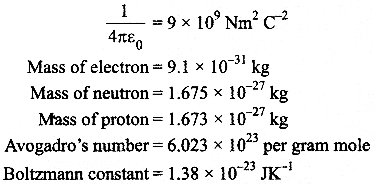Questions
SECTION : A

Question 1.
Show graphically, the variation of the de-Broglie wavelength (λ) with the potential (V) through which an electron is accelerated from rest.

Question 2.
A resistance R is connected across a cell of emf e and internal resistance r. A potentiometer now measures the potential difference between the terminals of the cell as V. Write the expression for V in terms of ε, V and R.

Question 3.
A plane electromagnetic wave travels in vacuum along Z-direction. What can you say about the direction of electric and magnetic field vector?

Question 4.
A point charge is placed at point O as shown in the figure. Is the potential difference VA-VB positive, negative or zero, if Q is
(i) positive
(ii) negative?Question 5.
The permeability of magnetic material is 0.9983. Name the type of magnetic materials it represents.

SECTION : B

Question 6.
Two uniformly large parallel thin plates having charge densities +σ and -σ are kept in the X- Z plane at a distance ‘d’ apart. Sketch an equipotential surface due to electric field between the plates. If a particle of mass m and charge ‘-q’ remains stationary between the plates, what is the magnitude and direction of this field?

OR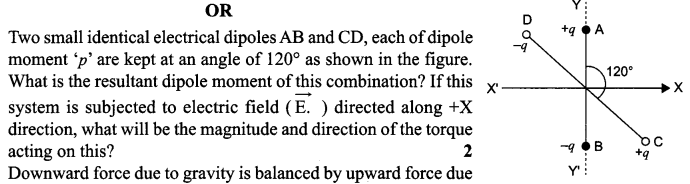Question 7.
Two convex lenses of same focal length but of aperture A1 and A2 (A2 < A1), are used as the objective lenses in two astronomical telescopes having identical eyepieces. What is the ratio of their resolving power? Which telescope will you prefer and why? Give reason.

Question 8.
A magnetic needle free to rotate in a vertical plane parallel to the magnetic meridian has its north tip down at 60° with the horizontal. The horizontal component of the earth’s magnetic field at the place is known to be 0.4 G. Determine the magnitude of the earth’s magnetic field at the place.

Question 9.
Figure shows two identical capacitors, C1 and C2, each of 1μF capacitance connected to a battery of 6 V. Initially switch ‘S’ is closed. After sometime ‘S’ is left open and dielectric slabs of dielectric constant K = 3 are inserted to fill completely the space between the plates of the two capacitors.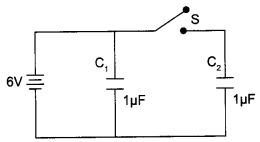How will the
(i) charge and
(ii) potential difference between the plates of the capacitors be affected after the slabs are inserted?

Question 10.
Draw the output waveform at X, using the given inputs A and B for the logic circuit shown below. Also, identify the logic operation performed by this circuit.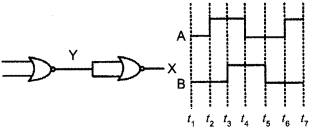Question 11.
Draw the transfer characteristic curve of a base biased transistor in CE configuration. Explain clearly how the active region of the V0 versus Vi– curve in a transistor is used as an amplifier.

Question 12.
(i) Define modulation index.
(ii) Why is the amplitude of modulating signal kept less than the amplitude of carrier wave?

Question 13.
A current is induced in coil C1due to the motion of current carrying coil C2.
(a) Write any two ways by which a large deflection can be obtained in the galvanometer G.
(b) Suggest an alternative device to demonstrate the induced current in place of a galvanometer.Question 14.
Define the terms
(i) drift velocity,
(ii) relaxation time. A conductor of length L is connected to a dc source of emf ε. If this conductor is replaced by another conductor of same material and same area of cross-section but of length 3L, how will the drift velocity change?

Question 15.
Name the semiconductor device that can be used to regulate an unregulated dc power supply. With the help of I-V characteristics of this device, explain its working principle.

Question 16.
Draw a schematic diagram showing the
(i) ground wave
(ii) sky wave and
(iii) space wave propagation modes for em waves.
Write the frequency range for each of the following :
(ii) Television
(iii) Satellite communication

Question 17.
How are infrared waves produced? Why are these referred to as ‘heat wave’? Write their one important use.

Question 18.
Using Gauss’s law obtain the expression for the electric field due to a uniformly charged thin spherical shell of radius R at a point outside the shell. Draw a graph showing the variation of electric field with r, for r > R and r < R.

Question 19.
An electron and a photon each have a wave lenght of 1.00 nm.
Find
(a) their momenta,
(b) the energy of the photon and
(c) the kinetic energy of electron

Question 20.
Describe Young’s double slit experiment to produce interference pattern due to a monochromatic source of light. Deduce the expression for the fringe width.

OR

Use Huygens principle to verify the laws of refraction.

Question 21.
(a) Describe briefly, with the help of suitable diagram, how the transverse nature of light can be demonstrated by the phenomenon of polarization.
(b) When unpolarized light passes from air to a transparent medium, under what condition does the refracted light get polarized?

Question 22.
The energy levels of a hypothetical atom are shown. Which of the shown transitions will result in the emission of a photon of wavelength 275 nm? Which of these transitions correspond to emission of radiation of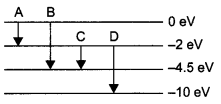(i) maximum and
(ii) minimum wavelength?

SECTION : D

Question 23.
In the circuit shown, R1 = 4 Ω, R2 = R3 = 15 Ω, R4 = 30Ω and E= 10 V. Calculate the equivalent resistance of the circuit and the current in each resistor.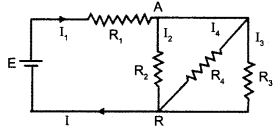SECTION : E

Question 24.
(i)  With the help of a labelled diagram, describe briefly the underlying principle and working of a step up transformer.
(ii) Write any two sources of energy loss in a transformer.
(iii) A step up transformer converts a low input voltage into a high output voltage. Does it violate law of conservation of energy? Explain.

OR

Derive an expression for the impedance of a series LCR circuit connected to an AC supply of variable frequency. Plot a graph showing variation of current with the frequency of the applied voltage. Explain briefly how the phenomenon of resonance in the circuit can be used in the tuning mechanism of a radio or a TV set.

Question 25.
State Biot-Savart law, giving the mathematical expression for it. Use this law to derive the expression for the magnetic field due to a circular coil carrying current at a point along its axis. How does a circular loop carrying current behave as a magnet?

OR

With the help of a labelled diagram, state the underlying principle of a cyclotron. Explain clearly how it works to accelerate the charged particles. Show that cyclotron frequency is independent of energy of the particle. Is there an upper limit on the energy acquired by the particle? Give reason.

Question 26.
(a) Draw a ray diagram to show refraction of a ray of monochromatic light passing through a glass prism.Deduce thee xpression for the refractive index of glass in terms of angle of prism and angle of minimum deviation.
(b) Explain briefly how the phenomenon of total internal reflection is used in fibre optics.

OR

(a) Obtain Lens Maker formula using the expressionHere the ray of light propagation from a rarer medium of refractive index (n1) to a denser medium of refractive index (n2) is incident on the convex side of spherical refracting surface of radius of curvature R.
(b) Draw a ray diagram to show the image formation by a concave mirror when the object is kept between its focus and the pole. Using this diagram, derive the magnification formula for the image found.

SECTION : A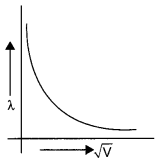In electromagnetic wave, the electric field vector E and magnetic field vector B show their variations perpendicular to the direction of propagation of wave as well as perpendicular to each other. As the electromagnetic wave is travelling along Z-direction, hence E and B show their variation in XY-plane.

If Q is positively charged, VA-VB = positive. If Q is negatively charged, VA-VB = negative.

Paramagnetic material (0 < μ < 1).

SECTION : B

Downward force due to gravity is balanced by upward force due to electric field.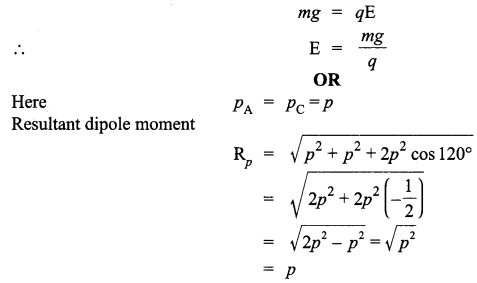When this dipole is placed in an electric field along + X-axis, it will experiences a torque.According to Right Hand Thumb Rule, torque will be into the plane of the paper.∴  The telescope with objective of aperture A1 should be preferred for viewing as this would :

1. give a better resolution.
2. have a higher light gathering power of telescope.

Given,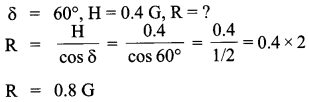When S is closed: Potential difference across C1  = Potential difference across C2 = 6V
V1 = V2 = 6V
∴ q1 = q2 = 1μF x 6V = 6μC
When S is open and dielectric slab (K = 3) are put in between plates of capacitors,
C= 3 x 1μF = 3μF
C= 3 x 1μF = 3μF
When S is open, potential difference across C1 , V1= 6V
∴ q1 = 3μF x 6V = 18μC
Potential difference across C2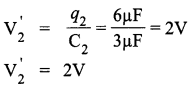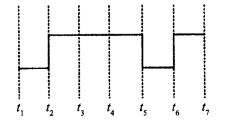The logic operation performed by this circuit is OR.

SECTION : C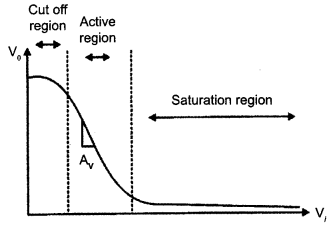In the active region, a (small) increase of Vi results in a (large, almost linear) increase in Ic. This results in an increase in the voltage drop across R.

(i) Modulation index is defined as the ratio of amplitude of modulating signal to amplitude of carrier wave. It is given by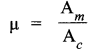Am = amplitude of modulation wave
Ac = amplitude of carrier wave
(ii) The amplitude of modulating signal is kept less than the amplitude of carrier wave to avoid noise.

(a) Any two ways to obtain large deflection in G :

• Moving C2 faster towards C1.
• Insertion of soft iron core in C1

(b) Alternative device that can be used in place of galvanometer is LED.

(i) Drift velocity :
The average velocity with which the free electrons drift towards positive terminal under the influence of an external field is called drift velocity.
(ii) Relaxation time :
Average time interval between two successive collisions of an electron with the ions/ atoms of the conductor.
The drift velocity will be inversely proportional to L, i.e.,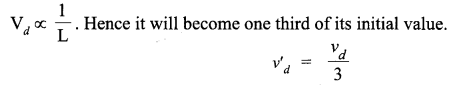A Zener diode is a specially designed diode which is operated in reverse breakdown region continuously with any damage. When Zener diode is operated in the reverse break down region, the voltage across it remains practically constant (Vz) for a large change in reverse current. Therefore, for any increase/ decrease of the input voltage there is a increase/ decrease of the voltage drop across series resistance without any change in the voltage across Zener diode,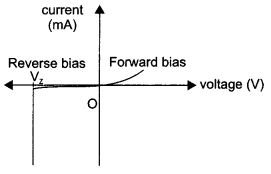(i) Standard AM Broadcast : 540-1600 kHz
(ii) Television : 54-72 MHz VHF (very high frequencies); 76-88 MHz TV; 174-216 MHz UHF (ultra high frequencies); 420-890 MHz TV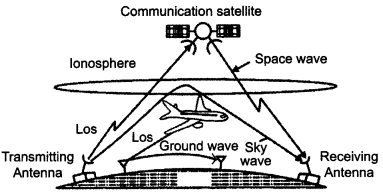Infrared rays are produced by hot bodies and molecules. Infrared waves are called heat waves as they cause heating effect. Infrared waves are used to maintain earth’s warmth, in physiotherapy, remote switches etc.

Electric field at point P at a distance r out side the spherical shell.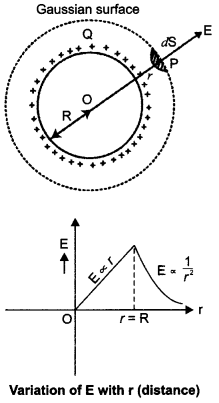According to gauss law,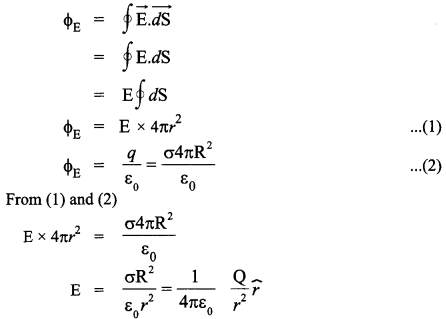image

λephoton = 1.00 nm= 10-9 m
(a) For electron or photon, momentum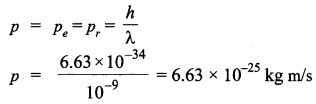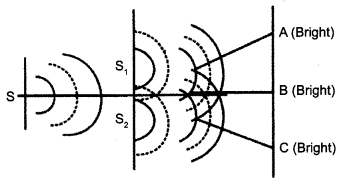S is a narrow slit (of width about 1 mm) illuminated by a monochromatic source of light. At a suitable distance (=10 mm) from S, two slits Sand S2 are placed parallel to S. When a screen is placed at a large distance (about 2m) from the slits Sand S2, alternate dark and bright bands appear on the screen. These are the interference bands or fringes. The band disappear when either slit is covered.
Explanation :
According to Huygens principle, the monochromatic source of light illuminating the slit S tends out spherical wavefront. The two waves of same amplitude and same frequency superimpose on each other, dark fringes appear on the screen when the crest of one wave falls on the trough of other and they neutralize the effect of each other. Bright fringes appear on the screen when the crest of one wave coincides with the crest of other and they reinforce each other.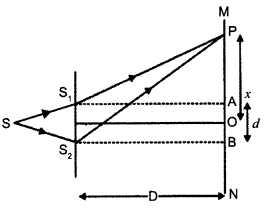Expression for the fringe width :
Let d = distance between slits S1 and S2
D = distance of screen from two slits and
x – distance between the central maxima
‘O’ and observation point P.
Light waves spread out from S and fall on both Sand S2. The spherical waves emanating from S1 and S2 will produce interference fringes on the screen MN.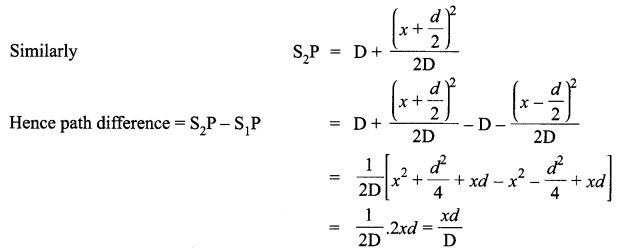Now, the intensity at point P is maximum or minimum accordingly as the path difference is an integral multiple of wavelength or an odd integral multiple of half wavelength.

OR

Wavefront :
The continuous locus of all the particles of a medium, which are vibrating in the same phase is called wavefront.
Laws of refraction :
Let PP’ represent the surface separating medium 1 and medium 2 as shown in fig.Which is Snell’s Law of refraction of light (second law)
First Law, incident wavefront, refracted wave front, normals all lie in the same plane.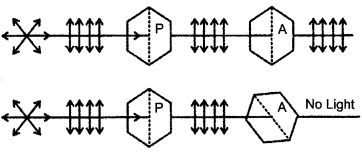(a) When a polaroid Pis rotated in the path of an unpolarised light, there is no change in transmitted intensity. The light transmitted through Polaroid P1 is made to pass through Polaroid P2. On rotating polaroid P2, in path of light transmitted from P1 we notice a change in intensity of transmitted light. This shows the light transmitted from P1 is polarized. Since light can be polarized, it has transverse nature.
(b) Whenever unpolarised light is incident from air to a transparent medium at an angle of incidence equal to polarizing angle, the reflected light gets fully polarized.If a photon of wavelength λ = 275nm is to be emited , they energy of photon is given byhence transition B would result in the emission of a photon of wavelenght 275nm.
(i) Transition A corresponds to max wavelenght due to least energy change transition.
(ii) Transition D corresponds to min wavelenght due to least energy change transition.

SECTION : D

R R3  R4  are in parallel combination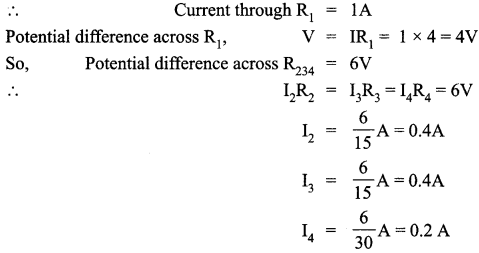SECTION : E

(i) Step-Up Transformer :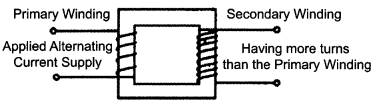Principle :

It works on the principle of mutual induction. When alternating current is passed through a coil an induced emf is set up in the neighbouring coil.
Working :
When an alternating voltage is applied to the primary, the resulting current produces an alternating magnetic flux which links the secondary and induces an emf in it. We consider an ideal transformer in which the primary has negligible resistance and all the flux in the core links with both primary and secondary windings. Let (Φ) be the flux in each turn in the core at any time t due to current in the primary when a voltage Vp is applied to it.
Then the induced emf or voltage Eç, in the secondary with Ns, turns is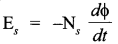The alternating flux also induces an emf, called back emf in the primary given by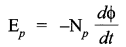But Ep = Vp (since resistance of primary is small) and Es. = Vs (since secondary current is small)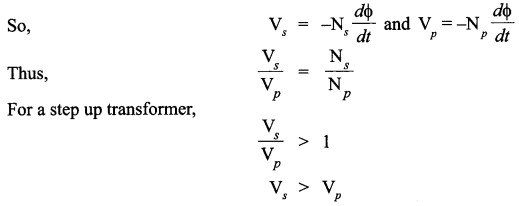(i) Sources of energy loss in transformer are : Heat loss due to resistance of windings, loss due to eddy currents.
(ii) A step up transformer steps up the voltage while it steps down the current. So the input and output power remain same (provided there is no loss). Hence there is no violation of the principle of energy conservation.

OR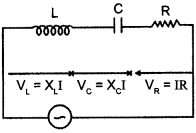The effective resistance offered by a series LCR circuit to the flow of current is called its impedance. It is denoted by Z. Suppose an inductance L, capacitance C and resistance R are connected in series to a source of alternating emf, V = V0 sin ωt
Let I be the instantaneous value of current in series circuit. Then voltages across the three components are

• VL = XL It is ahead of current I in phase by 90°
• Vc = XC It lags behind the current I in phase by 90°
• VR = RI. It is in phase with current I.

These voltages are shown in the phasor diagram given below :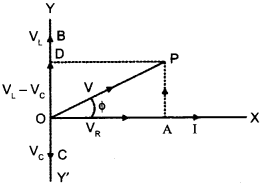As Vand Vc are in opposite direction, their resultant is OD = VL – Vc, in the positive y-direction.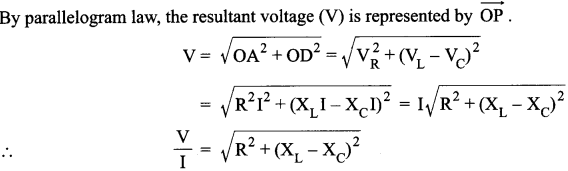Here, V/ I is the effective resistance of the series LCR circuit and is called its impedance (Z).The given graph showing variation of current with the frequency of the applied voltage. The radio and TV receiver sets are the practical applications of series resonant circuits. Signals of several different frequencies are available in air. By turning the tuning knob of the radio set, we vary the frequency of the LC circuit till it matches the frequency of the desired signal.

A series resonant circuit allows maximum current through it. So, it is called acceptor circuit. A series resonance circuit is that circuit in which inductance L, capacitance C and resistance R are connected in series. The impedance of this circuit has a minimum value and the current through the circuit is maximum.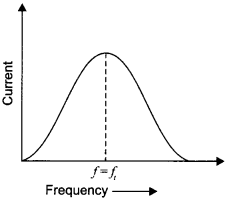Biot-Savart Law states that the magnitude of magnetic Y field dB due to current element is directly proportional to the current I,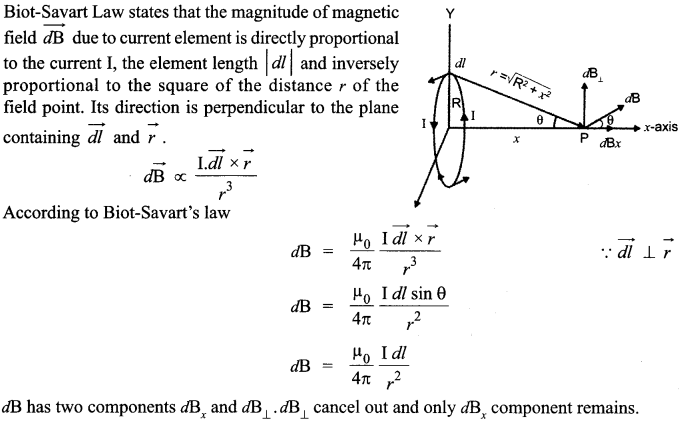Explanation :
A circular current loop produces magnetic field and its magnetic moment is the product of current and its area.
M = IA

OR

Principle :
Acharged particle can be accelerated to high energy by making it cross the same electric field again and again using a perpendicular magnetic field.Working :
High frequency oscillator maintains moderate alternating potential difference between the dees. This potential difference establishes an electric field that reverse its direction periodically. Suppose a positive ion of moderate mass produced at the centre of the dees, finds D2 at negative potential.It gets accelerated towards it. A uniform magnetic field, normal to the plane of the dees, makes it move in a circular track. Particle traces a semicircular track and returns back to the region between the dees. The moment it arrives in the region electric field reverses its direction and accelerate the charge towards D1 This way charge keeps on getting accelerated until it is removed out of the dees. Centripetal force, needed by the charged particle to move in circular track, is provided by the magnetic field.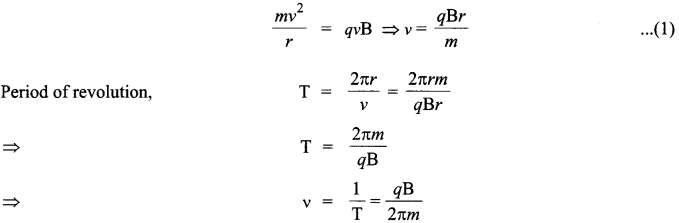Thus, frequency of revolution is independent of the energy of the particle. Yes, there is an upper limit on the energy acquired by the charged particle. The charged particle gains maximum speed when it moves in a path of radius equal to the radius of the dees.